# Leontief Model

## Definition

A Leontief Model is an economic model following the proposals of economist Wassily Leontief who developed a system of economic analysis (Input-Output Model) in the 1930s and 1940s. It is based on a linear economy assumption and demand-style economic modeling

## Formula

Starting with the market balance or Total Output equation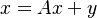$x = A x + y$

where

the Leontief inverse matrix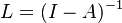$L = (I-A)^{-1}$ is obtained, satisfying the condition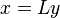$x = L y$.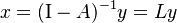\begin{align} x = (\mathrm{I}- A)^{-1}y = L y \end{align}

with$\mathrm{I}$ defined as the identity matrix (Kronecker delta) with the size of A.

## Interpretation

In the demand-driven, upstream or Leontief model, a matrix of direct requirements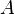$A$ is defined as the inter-industrial flows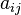$a_{ij}$ from an industry i to an industry j per gross output of sector j.

An element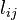$l_{ij}$ of the total requirements matrix, or Leontief inverse, L represents the amount of gross output from sector i that was produced to satisfy a unit of final demand y from sector j.

## Usage

An important goal of IO analysis is to examine the interdependencies between production and consumption within an economy. Such analysis includes the flow of goods of services between the economy and the rest of the world and can be expressed in monetary or other measurement units.